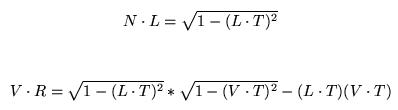Anisotropic Lighting(各向异性光照)是一种模拟有大量细小齐整沟槽（grooves）的表面的光照情况的图形学技术。在这种表面，光产生的效果是普通的图形学光照模型所无法模拟的。——ZwqXin.com（from Per-Pixel Strand Based Anisotropic Lighting（from NV SDK Anisotropic Lighting

1. //www.ZwqXin.com  Anisotropic lighting
2.
4. uniform vec4 lightpos;
5. uniform vec4 eyepos;
6. varying vec2 texCoord0;
7.
8. void main(void)
9. {
10.    vec3 norm = normalize(gl_NormalMatrix * gl_Normal);
11.
12.    vec4 pos = gl_ModelViewMatrix * gl_Vertex;
13.      vec3 vlightpos = (gl_ModelViewMatrix * lightpos).xyz;
14.    vec3 veyepos = (gl_ModelViewMatrix * eyepos).xyz;
15.
16.     vec3 vlightdir = normalize(vlightpos - pos.xyz);
17.     vec3 veyedir = normalize(veyepos - pos.xyz);
18.
19.     //h = normalize(l + e)
20.     vec3 halfVec = normalize(veyedir + vlightdir);
21.
22.     texCoord0.x = 2.0 * dot(halfVec, norm) - 1.0;//每个顶点的值随视线变化而变化
23.     texCoord0.y = 2.0 * dot(vlightdir, norm) - 1.0;//每个顶点拥有固定值
24.
25.    gl_Position = gl_ModelViewProjectionMatrix * gl_Vertex;
26. }
27.
29. uniform sampler2D tLookup;
30. uniform float intensity;
31. varying vec2 texCoord0;
32.
33. void main(void)
34. {
35.      vec4 col =  intensity * texture2D(tLookup, texCoord0.xy);
36.
37.    //gl_FragColor =  col; //1
38.    gl_FragColor =  vec4(col.rgb * (col.a), 1.0); //2
39. }

1. //Anisotropic Lighting 2  www.ZwqXin.com
2.
4. uniform vec4 baseColor;
5. uniform float controlR;
6.
7. uniform vec4 lightpos;
8. uniform vec4 eyepos;
9.
10. attribute vec3 rm_tangent;
11.
12. void main(void)
13. {
14.    vec3 norm = normalize(gl_NormalMatrix * gl_Normal);
15.    vec3 tang = normalize(gl_NormalMatrix * rm_tangent);
16.
17.    vec4 pos = gl_ModelViewMatrix * gl_Vertex;
18.    vec3 vlightpos = (gl_ModelViewMatrix * lightpos).xyz;
19.    vec3 veyepos = (gl_ModelViewMatrix * eyepos).xyz;
20.
21.     vec3 vlightdir = normalize(vlightpos - pos.xyz);
22.     vec3 veyedir = normalize(veyepos - pos.xyz);
23.
24.     //h = normalize(l + e)
25.     vec3 halfVec = normalize(veyedir + vlightdir);
26.
27. //0.3是光的波长，干脆就只用后面的调节系数controlR 调节好了
28.     float u = dot(tang, halfVec)*0.3；
29.     float w = dot(norm, halfVec);
30.
31.     float e = controlR * u / w;
32.     float c = exp(-e * e);
33.
34.     vec4 aniColor = baseColor * c;
35.
36.    gl_Position = gl_ModelViewProjectionMatrix * gl_Vertex;
37.    gl_FrontColor = aniColor;
38. }
39.
41. void main(void)
42. {
43.    gl_FragColor =  gl_Color;
44. }

•1.beyondborders
• 你好！我最近刚开始看glsl，你的文章让我学到很多东西。
我想问问你在Anisotropic lighting的实现代码中：
vec3 vlightpos = (gl_ModelViewMatrix * lightpos).xyz;
vec3 veyepos = (gl_ModelViewMatrix * eyepos).xyz;
这两句中lightpos，eyepos应该都是在world space里面指定，而不是object space中指定的吧？那么直接用gl_ModelViewMatrix左乘应该不会被转到eye space空间的吧？
zwqxin 于 2011-3-4 23:00:23 回复
是的，这里欠缺仔细的考虑了。THX指出。
• 2011-3-4 22:24:38 回复该留言

◎欢迎参与讨论，请在这里发表您的看法、交流您的观点。